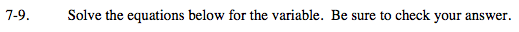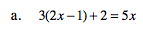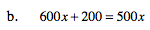### Home > MC2 > Chapter Ch7 > Lesson 7.1.1 > Problem7-9

7-9.Distrubute.

Combine like terms.

Subtract 5x from each side.

3(2x) − 3(1) + 2 = 5x

6x − 3 + 2 = 5x

6x − 1 = 5x

−5x + 6x − 1 = 5x −5x
x − 1 = 0

x − 1 = 0 +1 +1

x = 1Divide each term by 100.